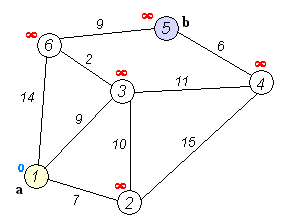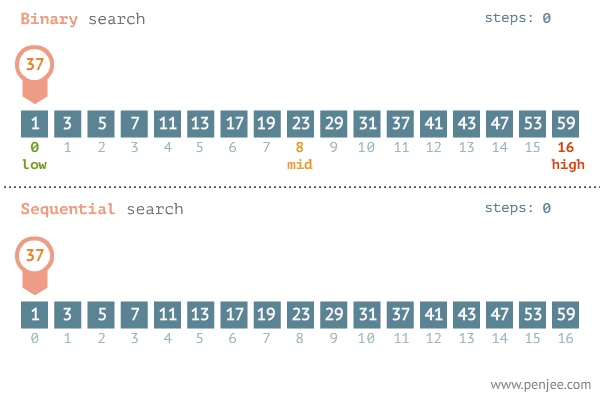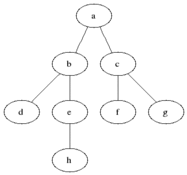I see many CS students get overwhelmed with the content that is on Internet today and often get confused on where to start this wonderful journey of becoming a master Computer Scientist.
I present in front of you some algorithms that are very important to start with.
These algorithms form the base of many advanced algorithms and are important for competitive coding.
These algorithms are also very frequently asked in tech interviews as they can be directly applied to many real world problems.
So let's dive in.

## 1. Dijkstra's AlgorithmDijkstra's Algorithm is a single source shortest path algorithm.
This algorithm is mostly used on graph data structures.
Given a graph and a source node, this algorithm gives us shortest paths from source to all the other nodes.
This algorithm is very frequently asked in tech interviews as it can be applied to many real life problems.

## 2. Binary SearchIt is one simple looking yet very powerful algorithm.
Given a sorted array and an element to find in that array, binary search performs the search in O(log n) time.
It is a significant improvement over the naive Linear Search algorithm which takes O(n) time to search an element. (To know more about time complexities, refer here)
The only problem with it is that it requires a sorted array to perform search.
Despite of this, it is very powerful as most of the real life data is sorted by some index.

1. Binary Search
2. Some Problems

## 3. Longest Common Subsequence

This is another very important algorithm that introduces us with the power of Dynamic Programming.
Dynamic Programming approach is a programming paradigm which is used to reduce the time complexity of several problems which contains Optimal Substructure and Overlapping Subproblems.
Instead of solving a part of problem again and again, it stores it in memory and checks this memory for solution before solving it again.
One very famous problem that can be solved by DP is Fibonnaci Sequence.

3. Fibonnaci Numbers

## 4. Graph Search AlgorithmsWhen we work with graphs, we should know how to manipulate them, populate them, and also search them.
Searching a graph is generally the first step towards solving most graph related problems.
So it is important to know how to search data in graphs.
Sometimes it is also called graph traversal.

We have broadly two categories for searching graphs

• Breadth First Search (BFS)- It searches the graph level by level.

• Depth First Search (DFS)- It searches the graph until the depth is covered.

These are the algorithms that form the base to all graph related problems.

## 5. Primality Tests

Checking whether a number is prime or not, and generating large prime numbers are classic problems in computer science.
A primality test checks whether a given number is prime or not.
Their are many different algorithms proposed by computer scientists but only some of are significance here.
Sieve of Eratosthenes is one of many primality tests and the most important one.

There are many more important and beautiful algorithms to work around, but this is a good place to start for any student who is new to Computer Science.
I will write more articles on important Data Structures and many more topics about programing so stay tuned!